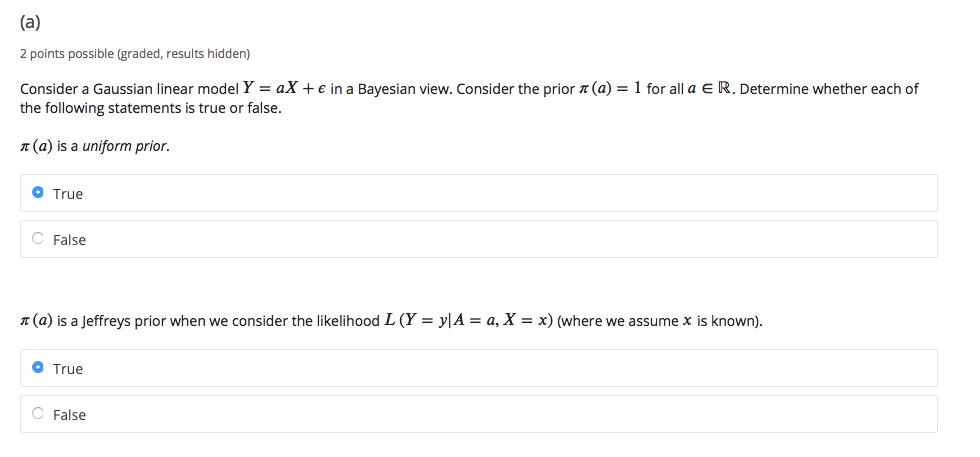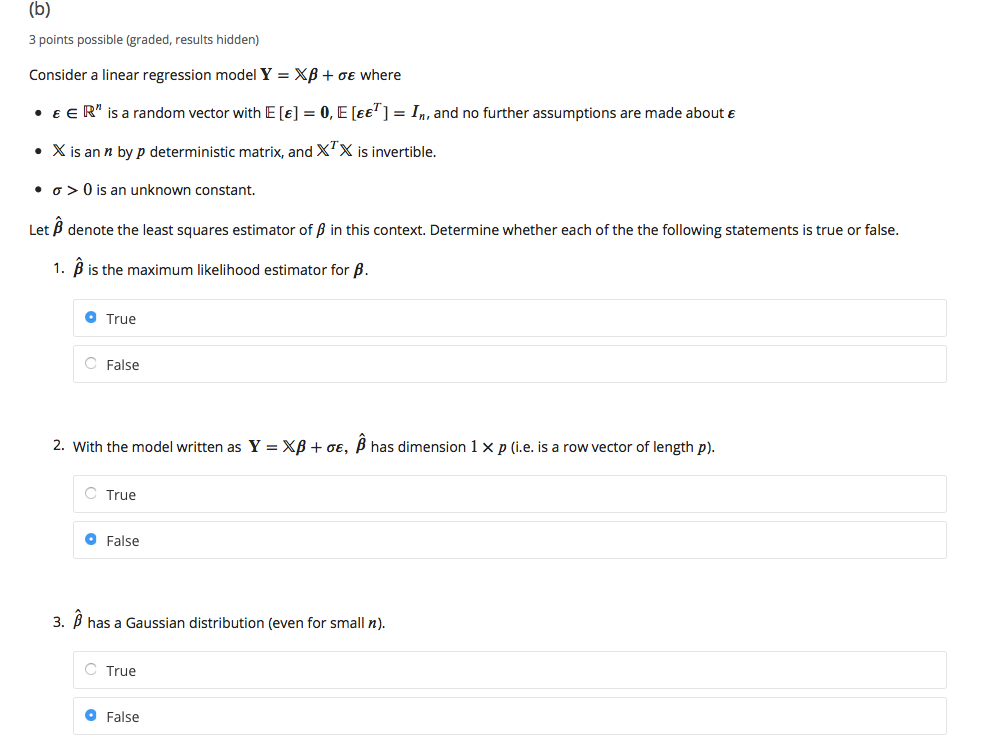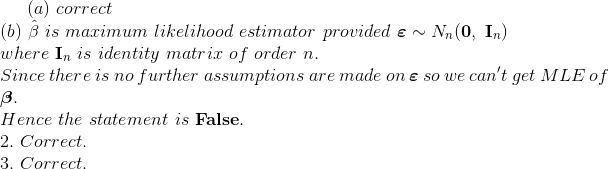# Do I get the right answers? If not, can someone please explain? (a) 2 points possible (graded, results hidden) Conside...

Do I get the right answers? If not, can someone please explain?(a) 2 points possible (graded, results hidden) Consider a Gaussian linear model Y = aX + e in a Bayesian view. Consider the prior (a) = 1 for all a eR. Determine whether each of the following statements is true or false. (a) is a uniform prior. O True C False n(a) is a Jeffreys prior when we consider the likelihood L (Y = y|A = a, X = x) (where we assume x is known). O True C False
(b) 3 points possible (graded, results hidden) Consider a linear regression model Y = Xß+ oe where E E R" is a random vector with E [e] = 0, E [ee' ] = I,n, and no further assumptions are made about e X is an n by p deterministic matrix, and X X is invertible. 0 is an unknown constant. O Let B denote the least squares estimator of B in this context. Determine whether each of the the following statements is true or false. 1. B is the maximum likelihood estimator for B. O True C False 2. With the model written as Y = XB + ae, B has dimension 1 xp (i.e. is a row vector of length p). True O False 3. В has a Gaussian distribution (even for small n). True O FalseMore Homework Help Questions Additional questions in this topic.